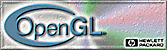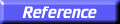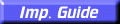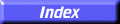# glRect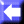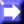glRectd, glRectf, glRecti, glRects, glRectdv, glRectfv, glRectiv, glRectsv: draw a rectangle.

C Specification | Parameters | Description | Errors | See Also

##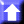C Specification

```void glRectd(
GLdouble	 x1,
GLdouble	 y1,
GLdouble	 x2,
GLdouble	 y2)
void glRectf(
GLfloat	 x1,
GLfloat	 y1,
GLfloat	 x2,
GLfloat	 y2)
void glRecti(
GLint	 x1,
GLint	 y1,
GLint	 x2,
GLint	 y2)
void glRects(
GLshort	 x1,
GLshort	 y1,
GLshort	 x2,
GLshort	 y2)
void glRectdv(
const GLdouble *v1,
const GLdouble *v2)
void glRectfv(
const GLfloat *v1,
const GLfloat *v2)
void glRectiv(
const GLint *v1,
const GLint *v2)
void glRectsv(
const GLshort *v1,
const GLshort *v2)
```

##Parameters

x1, y1
Specify one vertex of a rectangle.
x2, y2
Specify the opposite vertex of the rectangle.
v1
Specifies a pointer to one vertex of a rectangle.
v2
Specifies a pointer to the opposite vertex of the rectangle.

##Description

glRect supports efficient specification of rectangles as two corner points. Each rectangle command takes four arguments, organized either as two consecutive pairs of (x,y) coordinates, or as two pointers to arrays, each containing an (x, y) pair. The resulting rectangle is defined in the z = 0 plane.

glRect(x1, y1, x2, y2) is exactly equivalent to the following sequence:

```    glBegin(GL_POLYGON);
glVertex2(x1, y1);
glVertex2(x2, y1);
glVertex2(x2, y2);
glVertex2(x1, y2);
glEnd();
```
Note that if the second vertex is above and to the right of the first vertex, the rectangle is constructed with a counterclockwise winding.

##Errors

• GL_INVALID_OPERATION is generated if glRect is executed between the execution of glBegin and the corresponding execution of glEnd.

glBegin,
glVertex